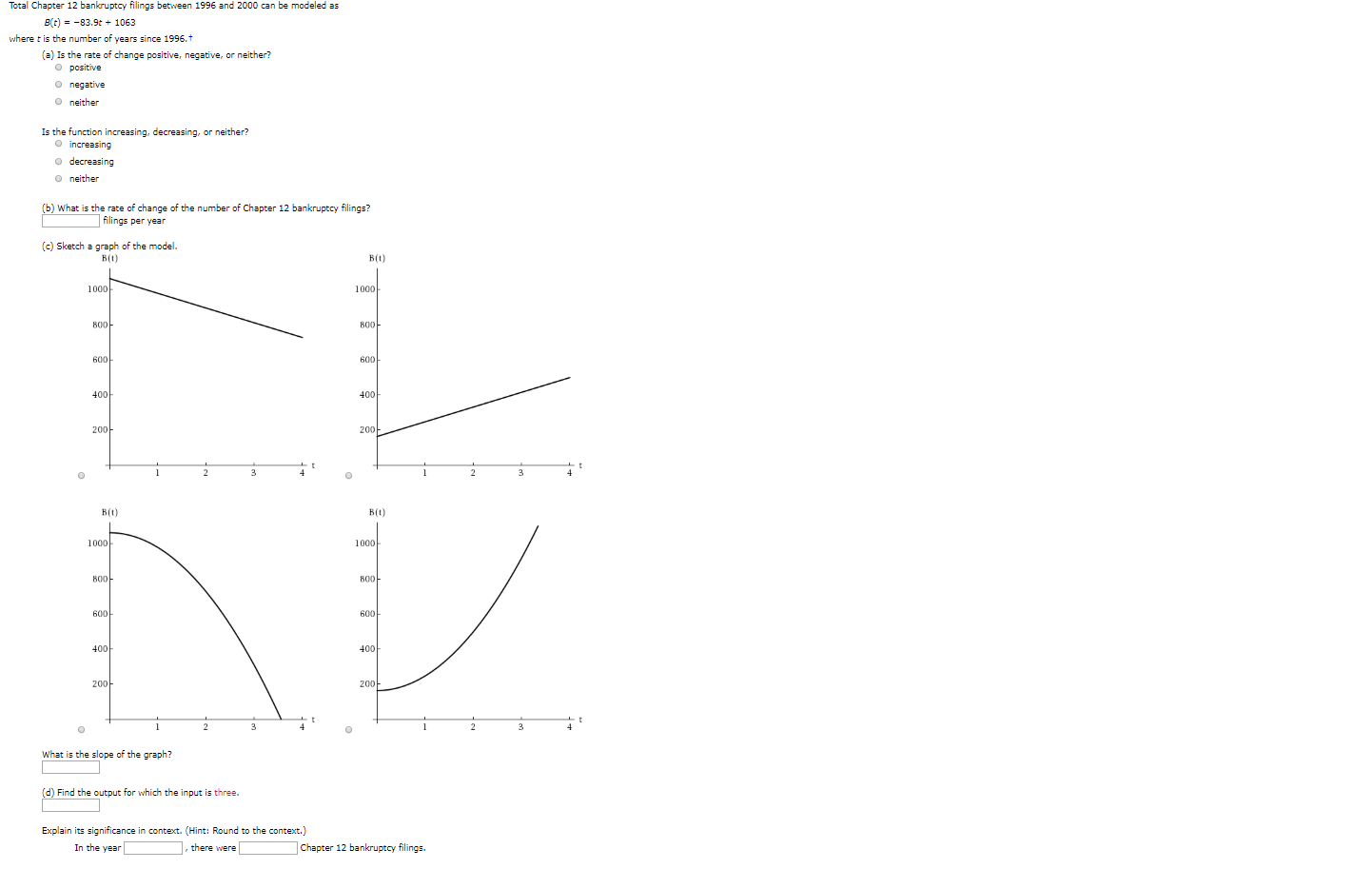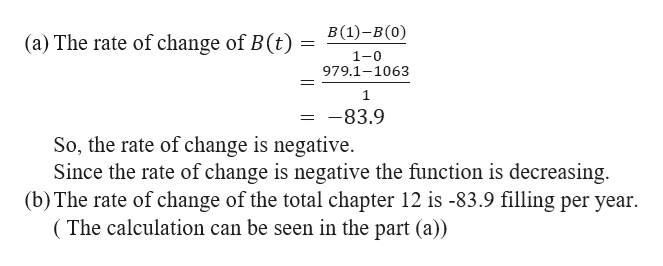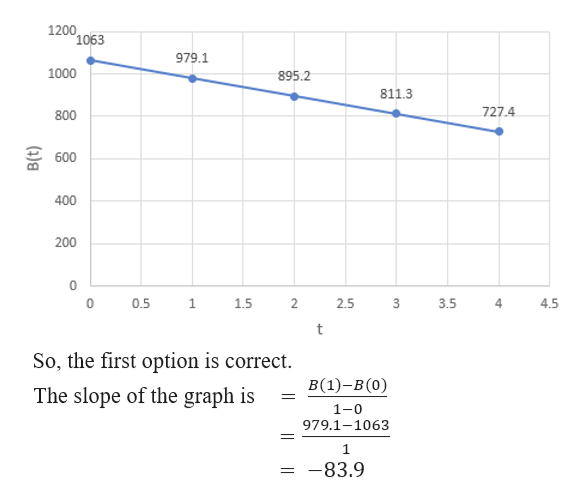Total Chapter 12 bankruptcy filings between 1996 and 2000 can be modeled asBt)-83.9t+ 1063where t is the number of years since 1996.t(a) Is the rate of change positive, negative, or neither?O positiveonegativeO neitherIs the function increasing, decreasing, or neither?O increasingo decreasingO neither(b) What is the rate of change of the number of Chapter 12 bankruptcy filings?filings per year(c) Sketch a graph of the model.B(tВ(0)10001000800800600600400400200200144CВ(0)В(0)100010008008006006004004002002002342CWhat is the slope of the graph?(d) Find the output for which the input is three.Explain its significance in context. (Hint: Round to the context.)there wereIn the yearChapter 12 bankruptcy filings.

Questionhelp_outlineImage TranscriptioncloseTotal Chapter 12 bankruptcy filings between 1996 and 2000 can be modeled as Bt)-83.9t+ 1063 where t is the number of years since 1996.t (a) Is the rate of change positive, negative, or neither? O positive onegative O neither Is the function increasing, decreasing, or neither? O increasing o decreasing O neither (b) What is the rate of change of the number of Chapter 12 bankruptcy filings? filings per year (c) Sketch a graph of the model. B(t В(0) 1000 1000 800 800 600 600 400 400 200 200 1 4 4 C В(0) В(0) 1000 1000 800 800 600 600 400 400 200 200 2 3 4 2 C What is the slope of the graph? (d) Find the output for which the input is three. Explain its significance in context. (Hint: Round to the context.) there were In the year Chapter 12 bankruptcy filings. fullscreen
Step 1

The total chapter 12 bankruptcy filings between 1996 and 2000 is modeled as B(t) = -83.9t + 1063, where t is the number of years since 1996. So, the values of t are 0 for 1996, 1 for 1997, 2 for 1998, 3 for 1999 and 4 for 2000.

Step 2

Here,help_outlineImage TranscriptioncloseВ (1)-в (0) (a) The rate of change of B(t) 1-0 979.1-1063 1 1 -83.9 So, the rate of change is negative. Since the rate of change is negative the function is decreasing (b) The rate of change of the total chapter 12 is -83.9 filling per year (The calculation can be seen in the part (a)) fullscreen
Step 3

(c)  Now, we need to draw the...help_outlineImage Transcriptionclose1200 1063 979.1 1000 895.2 811.3 727.4 800 600 400 200 0 0.5 1 1.5 2 2.5 3.5 4.5 t So, the first option is correct B(1)-B (0) The slope of the graph is 1-0 979.1-1063 -83.9 fullscreen

Want to see the full answer?

See Solution

Want to see this answer and more?

Our solutions are written by experts, many with advanced degrees, and available 24/7

See Solution
Tagged in

Functions